# Fraction Math

The fraction math app can be used to teach and study fractions. It is the only fraction math app in the App Store that allows the user to solve random and custom fraction problems step by step.

The Fraction Math app can handle addition, subtraction, multiplication and division of positive and negative fractions, improper fractions, whole numbers and mixed numbers. The user can also reduce the resulting fractions.

##Suitable for several grades

A third-grade student can use the app to add and subtract fractions with equal denominators.

A fourth-grade student can use the app to add or subtract fractions and mixed numbers with different denominators and also to reduce the resulting fractions.

A fifth-grade student can multiply fractions by whole numbers and other fractions. She can also divide fractions and mixed numbers.

A sixth or higher-grade student can use negative fractions, whole numbers and mixed numbers in the problems. She can also use larger numbers with the problems.

The above grades and their uses of the app are only provided as examples. Introduction of different mathematical concepts can vary in different states and countries.

The user can also see the problem and the answer in decimal notation, if she wants to.

## The user can choose how to solve the problems

In each step the user is presented with two choices. Sometimes both of the choices are right. When multiplying fractions the user can choose to first multiply the numerators or the denominators.

In those cases when there is only one possible next step, the user can choose between the right step and a random wrong step. If the user chooses the wrong step, the app will tell, why that is the wrong step. In adding a whole number and a fraction the whole number must be written as a fraction first.

The help system provides additional information in all steps.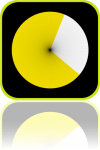Please also see the Visual Fractions Decimals and Percentages app for building conceptual understanding.

## Intelligent help system

The Fraction Math app has a help system that provides over 60 context sensitive tips based on the state of the app. For example, when searching for a lowest common denominator, the help system will first tell how to search for the answer. If, after trying herself the user cannot find the answer, the help system will tell her what the lowest common denominator is. If the user finds a common denominator that is not the lowest common denominator, the help system will note this but will let the user to continue. She will just have to reduce the answer at the end.

## Smart keyboard

When the user has to enter numbers, the Fraction Math app uses the same intuitive smart rolling keyboard as other apps by the developer. Only one tap will be needed to enter any number.

In reducing fractions, a special prime number keyboard is shown.

## Every step is animated

Every step in solving the problems is animated. In dividing two fractions the second fraction is first inverted by turning it upside down. The inverting is animated. The speed of the animations can be set and they can also be turned off.

## Three different themes

The app has three different themes. The Happy theme has illustrations. If the user prefers a theme without illustrations there is also a black and a white theme.

## Difficulty can be set with Settings

• Allow subtraction
• Allow multiplication
• Allow division
• Only allow fractions with same denominators
• Allow whole numbers
• Allow mixed numbers
• Allow negative numbers
• Allow numbers greater than 12

## Fraction Math Videos

#### Adding fractions with different denominators#### Multiplying fractions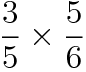#### Dividing fractions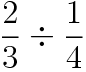#### Subtracting fractions with same denominators#### Fraction division with mixed numbers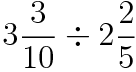#### Fraction addition with mixed number and whole number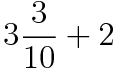## Fraction Math in the Apple VPP Store for Education

The Volume Purchase Program allows participating educational institutions to purchase iDevBooks math apps in volume and distribute them to students. All iDevBooks math apps offer special 50% discount for purchases of 20 apps or more for participating educational institutions.

Fraction Math in the VPP store